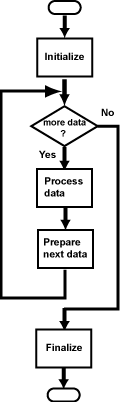Yes. When ever something is done repeatedly there is a loop (or recursion.)

# What Kind of Loop?If there is a loop (and 99% of the time there is), then the program will almost certainly follow the universal flow chart.

Now the problem is: What kind of loop? The user is expected to enter values for `raw` one by one until the loop is done. But how does the loop end? There are three kinds of loops:

• Counting loops, that execute the loop body a number of times known before the loop begins.
• Sentinel loops, where a special value in the input data signals the end of the loop.
• Result-controlled loops, that keep looping until the computation has reached a particular goal without knowing how long that might take.

A counting loop would require the user to first enter the number of raw scores. This is possible, but awkward, since now the user must count values. This would be unpleasant if there were hundreds of values.

A sentinel loop would require the user to enter a special value to signal the end of data. Another possibility that the user is asked if there is more data before each iteration of the loop. Logically this is fine, but it doubles the amount of time spent on entering data.

A result-controlled loop is used when all the values entered in the loop are used as a group to calculate something that depends on them all. This is not the case here.

### QUESTION 3:

What type of loop do you propose for this program?

Hint: Raw scores are in the range 0 to 300.